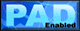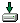# WebCab Probability and Stat (J2EE Ed.) 3.3EJB Suite offers functionality from Basic Statistics, Discrete Probability, Standard Probability Distributions, Hypothesis Testing, Correlation and Linear Regression. Statistics Module The Statistics module incorporates evaluation procedures of standard quantitative measures of centrality (mean) and dispersion of (discrete) numerical sets. This module incorporates weighted averages, geometric mean, Inter-Quartile range, mean and standard deviation, sample variance and the coefficient of variation. Discrete Probability Module The Discrete Probability module encapsulates the foundations of discrete probability and discrete probability distributions. This component includes the addition law, conditional probability, cumulative distribution function, mean and variance of a distribution, expected values, covariance and simplification of expressions involving random variables. Correlation and Regression Module Allows the user to investigate relationships between two variables. These finding can be used to predict one variable from the given values of other variables. We cover linear (Spearman's, t-test, z-transform) and rank (Spearman's, Kendall's) correlation, linear regression and conditional means. Standard Probability Distributions Module This module assists in the development of applications that incorporate the Binomial, Poisson, Normal, Lognormal, Pareto, Uniform, Hypergeometric and Exponential probability distributions. The probability density function, cumulative distribution function and inverse, mean, variance, Skewness and Kurtosis are implemented where appropriate and/or their approximations for each distribution. We also offer methods which randomly generate numbers from a given distribution. Confidence Intervals and Hypothesis Testing Module Within this component we present two aspects of inferential statistics known as confidence intervals and hypothesis testing.
 Version:  3.3 Release Date:  10/05/2004 Size:  20129  KB Platform:  Win98,Windows2000,WinXP,Windows2003,Unix,Linux Program type:  CommercialCost: \$249Online order: purchaseReview: Click here
 Requirements:  A J2EE1.3 (EJB2.0) compatible Application Server